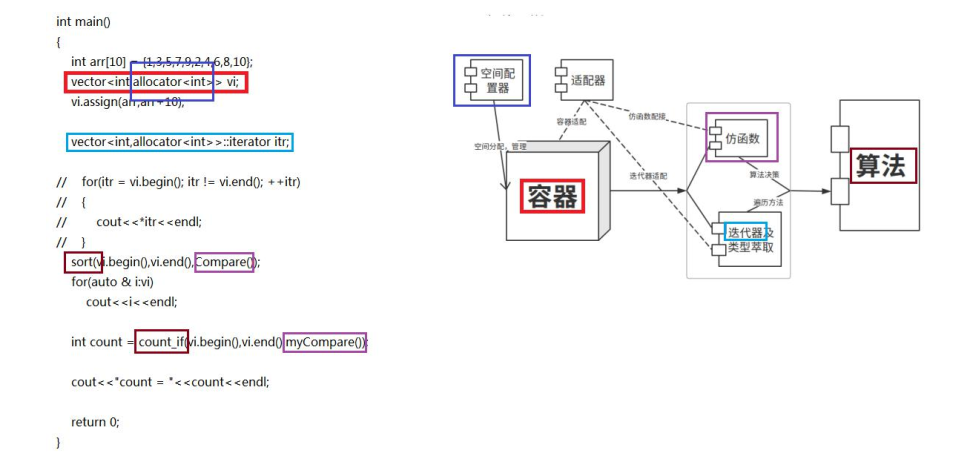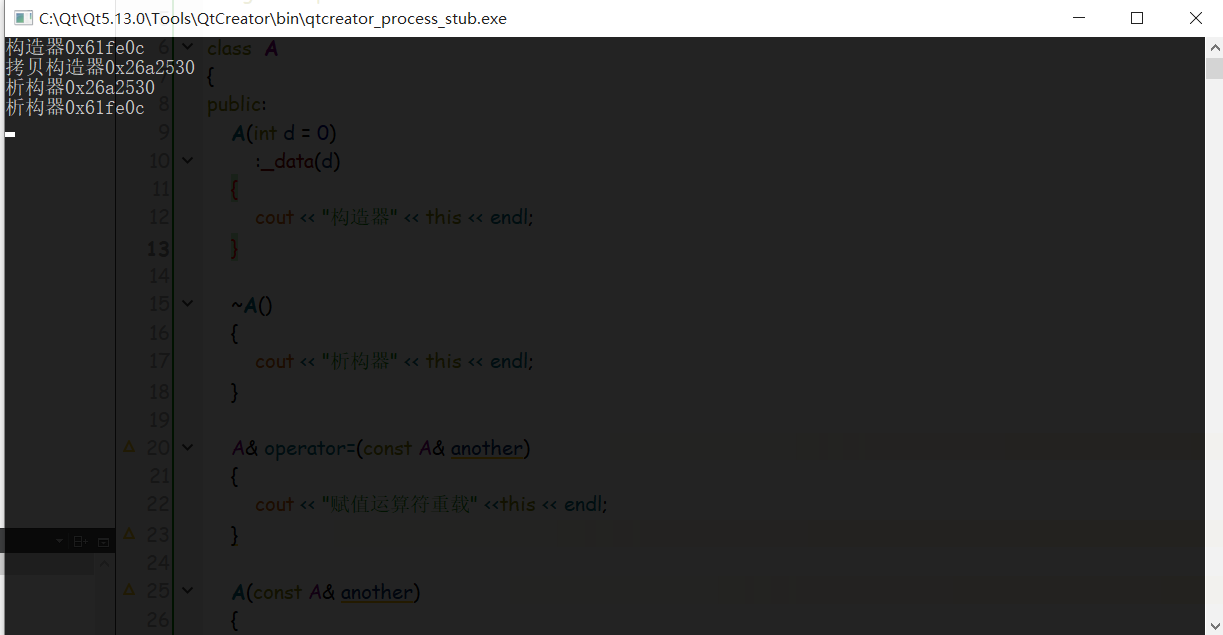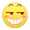# STL入门

## 1、STL是什么

STL = Standard Template Library，是一个函数库。STL 是由 C++模板编写的一套己纳入 c++标准的类库， 该库解决了通用数据结构与算法的问题。

STL 它是由 Alexander Stepanov、 Meng Lee 和 David R Musser 在惠普实验室工作时所开发出来的。亚历山大·斯特潘诺夫(AlexanderStepanov)——STL(标准模板库)之父， 并因此而荣获第一届 Dr. Dobb's 程序设计杰出奖， 现在是 Adobe 公司首席科学家。

STL源码地址链接在这里

## 2、STL的一个小程序示例

``````#include <iostream>
#include <vector>
#include <algorithm>
#include <functional>//bind需要这个头文件

using namespace std;

class  compare
{
public:
bool operator()(int x , int y)
{
return x>y;
}
};

int main()
{
int arr =  {1,2,3,4,8,6,9};
vector<int> vi ;
vi.assign(arr, arr+7);
vector<int>::iterator itr;
for(itr=vi.begin() ; itr != vi.end() ; ++itr)
{
cout<< *itr << endl;
}
cout << "------------------------------------" << endl;
sort(vi.begin(),vi.end(),compare());  //仿函数匿名对象
for(itr=vi.begin() ; itr != vi.end() ; ++itr)
{
cout<< *itr << endl;
}
cout << "------------------------------------" << endl;
int count = count_if(vi.begin(),vi.end(),bind(less<int>() , placeholders::_1,5));//bind绑定对象所用
int count = count_if(vi.begin(),vi.end(),bind(greater<int>() , placeholders::_1,5));//求大于5的数
cout << count << endl;
return 0;
}
``````

## 3、STL组成图示sort函数使用要包含`#include <algorithm>`算法库。

## 4、容器的特性

### 特点2：保存副本

``````#include <iostream>
#include <vector>

using namespace std;

class  A
{
public:
A(int d = 0)
:_data(d)
{
cout << "构造器" << this << endl;
}

~A()
{
cout << "析构器" << this << endl;
}

A& operator=(const A& another)
{
cout << "赋值运算符重载" <<this << endl;
}

A(const A& another)
{
cout << "拷贝构造器" << this <<endl;
}
private:
int _data;
};

int main()
{
A a;
vector<A> va;
va.push_back(a);//注意此句话
return 0;
}``````## 5、容器适用场景SWOT分析

VectorDequeListSetMultiSetMapMultiMap

Last modification：October 6th, 2019 at 02:20 pm1.正好最近在学这个